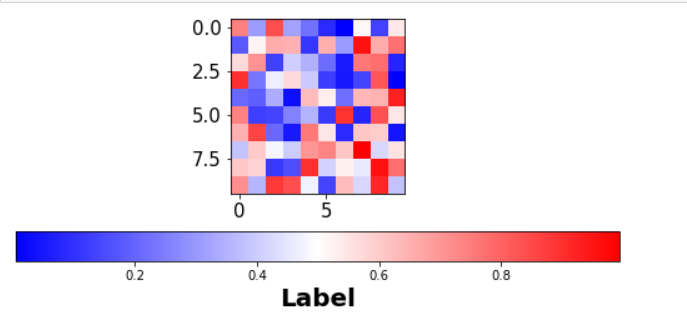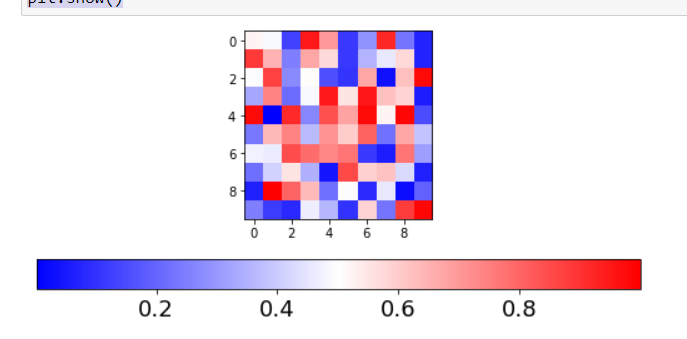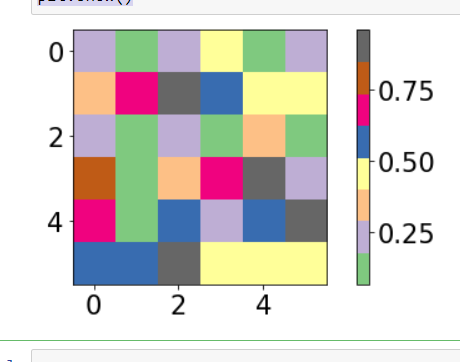Open in App
Not now

# Change the label size and tick label size of colorbar using Matplotlib in Python

• Last Updated : 05 Nov, 2021

In this article, we will learn how to change the label size and tick label size of colorbar in Matplotlib using Python.

Labels are a kind of assigning name that can be applied to any node in the graph. They are a name only and so labels are either present or absent. To properly label a graph, helps to identify the x-axis and y-axis. Each tick mark represents a specified value of units on a continuous scale or the value of a category on a categorical scale. The X-axis and the Y-axis are noted on the graph.

Here we will discuss how to change the label size and tick label size of color-bar, using different examples to make it more clear.

Syntax:

# Change the label size

im.figure.axes.tick_params(axis=”both”, labelsize=21)

• axis = x, y or both.
• labelsize = int

# Change the tick label size of color-bar

im.figure.axes.tick_params(axis=””, labelsize=21)

• axis = x, y or both.
• labelsize = int

Example 1: In this example, we are changing the label size in Plotly Express with the help of method im.figure.axes.tick_params(axis=”both”, labelsize=21), by passing the parameters axis value as both axis and label size as 21.

## Python3

 `# importing libraries``import` `numpy as np``import` `matplotlib as mpl``import` `matplotlib.pyplot as plt`` ` `# setup data``a ``=` `np.random.rand(``10``, ``10``)``im ``=` `plt.imshow(a, cmap``=``"bwr"``)``cb ``=` `plt.colorbar(im, orientation``=``'horizontal'``)`` ` `# change the label size``im.figure.axes[``0``].tick_params(axis``=``"both"``, labelsize``=``21``)`` ` `plt.show()`

Output:Example 2: In this Example, we are changing the label size in Plotly Express with the help of method im.figure.axes.tick_params(axis=”x”, labelsize=18), by passing the parameter axis value as x and label size as 18.

## Python3

 `# importing libraries``import` `numpy as np``import` `matplotlib as mpl``import` `matplotlib.pyplot as plt`` ` `# setup data``a ``=` `np.random.rand(``10``, ``10``)``im ``=` `plt.imshow(a, cmap``=``"bwr"``)``cb ``=` `plt.colorbar(im, orientation``=``'horizontal'``)`` ` `# change the tick label size of colorbar``im.figure.axes[``1``].tick_params(axis``=``"x"``, labelsize``=``18``)`` ` `plt.show()`

Output:Example 3: In this example, we are changing the label size in Plotly Express with the help of method im.figure.axes.tick_params(axis=”y”, labelsize=21), bypassing the parameter axis value as y and label size as 21.

## Python3

 `# importing libraries``import` `numpy as np``from` `matplotlib ``import` `pyplot as plt`` ` `# setup data``plt.rcParams[``"figure.figsize"``] ``=` `[``7.00``, ``3.50``]``plt.rcParams[``"figure.autolayout"``] ``=` `True``data ``=` `np.random.rand(``6``, ``6``)``im ``=` `plt.imshow(data, interpolation``=``"nearest"``, cmap``=``"Accent"``)``cbar ``=` `plt.colorbar(im)`` ` `# change the label size``im.figure.axes[``0``].tick_params(axis``=``"both"``, labelsize``=``21``)`` ` `# change the tick label size of colorbar``im.figure.axes[``1``].tick_params(axis``=``"y"``, labelsize``=``21``)`` ` `plt.show()`

Output:My Personal Notes arrow_drop_up